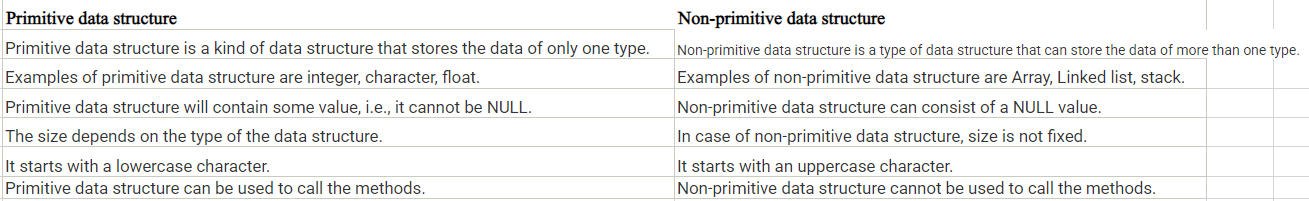Java Data-Types

# Java Data-Types

Java Datatypes
As we discussed earlier every variable must be a specified datatype.
Types of datatypes
In java data types are divided into two categories-
1. Primitive data types
2. Non- primitive data types
We will discuss both of the types in detail as follows-
1. Primitive datatypes
Primitive datatypes are predefined data types, and are also called built-in data types.
They are part of language, and Java has 8 fundamental detatyes.
Numeric data types-
Numeric data types are divided into two main categories defined as follows-
1.Integer Type
Integer type further divided into 4 different types-
i.Byte
This data type can store numbers from -128 to 127. This can be used instead of int when the given value is taking 1 byte of space.
 byte num =110; System.out.println(num);
ii.Short
This data type can store numbers from -32768 to 32767. This can be used instead of int when the inputting value in 2 byte space, or less.
 short num =4000; System.out.println(num);
iii. int
The int data type can store numbers from -2147483648 to 2147483647.The int data type is the preferred data type when we create variables with a numeric value, and when the space required by inputting value is 4 byte or less.
 int num =400000; System.out.println(num);
iv.long
The long data type can store whole numbers from -9223372036854775808 to 9223372036854775807. This is used when int is not large enough to store the value. Note that you should end the value with an "L".
 short num =400000000L; System.out.println(num);
2. Floating Point Types-
Floating point types are further divided into two types.
i.Float
You should use a floating point type whenever you need a number with a decimal, such as 3.14 or 32.1456. We use float data type when the space required is 4 byte or less, it the space requirement is more we use double , and put f in the end of value-
 float num =3.14f; System.out.println(num);
ii.Double
The double data type can store fractional numbers from 1.7e−308 to 1.7e+308. We use floating points when the space required is 8 byte or less.
 duble num =30.20d; System.out.println(num);
iii.Boolean
A boolean data type is declared with the boolean keyword and can only take the values true or false.
 boolean x=true; boolean a =false; System.out.println(x); System.out.println(a);
iv.Character
The char data type is used to store a single character. The character must be surrounded by single quotes, like ‘a’, ‘A’.
 char x= ‘a’ char a = ‘b’; System.out.println(x); System.out.println(a);
v.String
String is a data type used to store the sequence of characters. String values must be surrounded by double quotes like “web designing house”, “Online Promotion house ” etc.
 String num = “Web designing house”; String a = “Any text”; System.out.println(num); System.out.println(a);
2. Non-Primitive datatypes
Non- primitive data types are user defined data types, created by programmer like Array, class, interface..etc.
The main difference between primitive and non-primitive data types are-